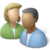## Spring Design Optimization Problem

The specifications and modeling equations for a compression spring create a number of trade-offs that must be considered during design. We wish to determine the spring design that maximizes the force of a spring at its preload height, ho, of 1.0 inches. The spring is to operate an indefinite number of times through a deflection deltao, of 0.4 inches, which is an additional deflection from ho. The stress at the solid height, hs, must be less than Sy to protect the spring from inadvertent damage.Turn in a report with the following sections:

1. Title Page with Summary. The Summary should be short (less than 50 words), and give the main optimization results.
2. Procedure: Give a brief description of your model. You are welcome to refer to the assignment which should be in the Appendix. Also include:
1. A table with the analysis variables, design variables, analysis functions and design functions.
3. Results: Briefly describe the results of optimization (values). Also include:
1. A table showing the optimum values of variables and functions, indicating binding constraints and/or variables at bounds (highlighted)
2. A table giving the various starting points which were tried along with the optimal objective values reached from that point.
4. Discussion of Results: Briefly discuss the optimum and design space around the optimum. Do you feel this is a global optimum? Also include and briefly discuss:
1. A “zoomed out” contour plot showing the design space (both feasible and infeasible) for coil diameter vs. wire diameter, with the feasible region shaded and optimum marked.
2. A “zoomed in” contour plot of the design space (mostly feasible space) for coil diameter vs. wire diameter, with the feasible region shaded and optimum marked.
5. Appendix:
1. Listing of your model with all variables and equations
2. Solver output with details of the convergence to the optimal values

Any output from the software is to be integrated into the report (either physically or electronically pasted) as given in the sections above. Tables and figures should all have explanatory captions. Do not just staple pages of output to your assignment: all raw output is to have notations made on it. For graphs, you are to shade the feasible region and mark the optimum point. For tables of design values, you are to indicate, with arrows and comments, any variables at bounds, any binding constraints, the objective, etc. (You need to show that you understand the meaning of the output you have included.)

#### Help SessionThis assignment can be completed in collaboration with others. Additional guidelines on individual, collaborative, and group assignments are provided under the Expectations link.

Solution Helpfrom gekko import GEKKO

# Initialize Gekko model
m = GEKKO()

#Maximize force of a spring at its preload height h_0 of 1 inches
#The stress at Hs (solid height) must be less than Sy to protect from damage

# Constants
from numpy import pi

# Model Parameters
delta_0 = 0.4 # inches (spring deflection)
h_0 = 1.0 # inches (preload height)
Q = 15e4 # psi
G = 12e6 # psi
S_e = 45e3 # psi
S_f = 1.5
w = 0.18

# Variables
# inches (wire diameter)
d_wire = m.Var(value=0.07247, lb = 0.01, ub = 0.2)
# inches (coil diameter)
d_coil = m.Var(value=0.6775, lb = 0.0)
# number of coils in the spring
n_coils = m.Var(value=7.58898, lb = 0.0)
# inches (free height spring exerting no force)
h_f = m.Var(value=1.368117, lb = 1.0)
F = m.Var() # Spring force

# Intermediates
S_y = m.Intermediate((0.44 * Q) / (d_wire**w))
h_s = m.Intermediate(n_coils * d_wire)
k = m.Intermediate((G * d_wire**4)/(8 * d_coil**3 * n_coils))
kW = m.Intermediate((4 * d_coil - d_wire)/(4 * (d_coil-d_wire)) \
+ 0.62 * d_wire/d_coil)
n = m.Intermediate((8 * kW * d_coil)/(pi * d_wire**3))
tau_max = m.Intermediate((h_f - h_0 + delta_0) * k * n)
tau_min = m.Intermediate((h_f - h_0) * k * n)
tau_mean = m.Intermediate((tau_max + tau_min) / 2)
tau_alt = m.Intermediate((tau_max - tau_min) / 2)
h_def = m.Intermediate(h_0 - delta_0)
tau_hs = m.Intermediate((h_f - h_s) * k * n)

# Equations
m.Equations([
F == k * (h_f - h_0),
d_coil / d_wire >= 4,
d_coil / d_wire <= 16,
d_coil + d_wire < 0.75,
h_def - h_s > 0.05,
tau_alt < S_e / S_f,
tau_alt + tau_mean < S_y / S_f,
tau_hs < S_y / S_f
])

# Objective function
m.Obj(-F)

# Send to solver
m.solve()

# Print solution
print('Maximum force: ' + str(F))
print('Optimal values: ')
print('d_wire : ' + str(d_wire))
print('d_coil : ' + str(d_coil))
print('n_coils : ' + str(n_coils))
print('h_f : ' + str(h_f))